# HBSE 7th Class Maths Solutions Chapter 2 Fractions and Decimals InText Questions

Haryana State Board HBSE 7th Class Maths Solutions Chapter 2 Fractions and Decimals InText Questions and Answers.

## Haryana Board 7th Class Maths Solutions Chapter 2 Fractions and Decimals InText Questions

Try These (Page 34):

Question 1.
If the product is an improper fraction express it as a mixed fraction.
Find
(a) $$\frac{2}{7}$$ x 3
(b) $$\frac{9}{7}$$ x 6
(c) 3 x $$\frac{1}{8}$$
(d) $$\frac{13}{11}$$ x 6
Solution: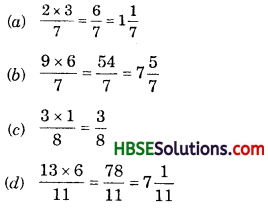Question 2.
Represent pictorially : 2 x $$\frac{2}{5}=\frac{4}{5}$$
Solution: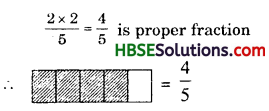Try These (Page 34):

Question 1.
Find
(i) 5 x 2$$\frac{3}{7}$$
(ii) 1$$\frac{4}{9}$$ x 6
Solution: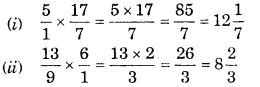Try These (Page 35):

Question 1.
Can you tell, what is
(i) $$\frac{1}{2}$$ of 10 = ?
(ii) $$\frac{1}{4}$$ of 16 = ?
(iii) $$\frac{2}{5}$$ of 25 = ?
Solution:
(i) $$\frac{1}{2}$$ of 10 = 5
(ii) $$\frac{1}{4}$$ of 16 = 4
(iii) $$\frac{2}{5}$$ of 25 = 2 x 5 = 10

Try These (Page 39):

Question 1.
Fill in these boxes :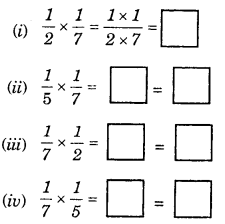Solution: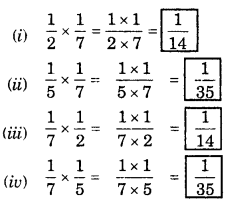Try These (Page 40):

Question 1.
Find
(i) $$\frac{1 \times 4}{3 \times 5}$$
(ii) $$\frac{2 \times 1}{3 \times 5}$$
Solution:
(i) $$\frac{1 \times 4}{3 \times 5}=\frac{4}{15}$$
(ii) $$\frac{2 \times 1}{3 \times 5}=\frac{2}{15}$$

Try These (Page 40):

Question 1.
Find
(i) $$\frac{8}{3} \times \frac{4}{7}$$
(ii) $$\frac{3}{4} \times \frac{2}{3}$$
Solution:
(i) $$\frac{8 \times 4}{3 \times 7}=\frac{32}{21}$$
(ii) $$\frac{3 \times 2}{4 \times 3}=\frac{2}{4}=\frac{1}{2}$$

Try These (Page 45):

Question 1.
Find
(i) 7 ÷ $$\frac{2}{5}$$
(ii) 6 ÷ $$\frac{4}{7}$$
(iii) 2 ÷ $$\frac{8}{9}$$
Solution: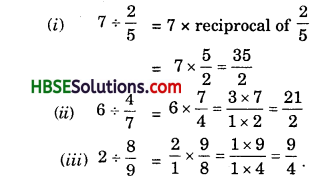Try These (Page 45):

Question 1.
Find
(i) 6 ÷ 5$$\frac{1}{3}$$
(ii) 7 ÷ 2$$\frac{4}{7}$$
Solution:
(i) $$\frac{6}{1} \div \frac{16}{3}=\frac{6}{1} \times \frac{3}{16}=\frac{3 \times 3}{1 \times 8}=\frac{9}{8}$$
(ii) $$7 \div \frac{18}{7}=7 \times \frac{7}{18}=\frac{49}{18}$$Try These (Page 45):

Question 1.
Find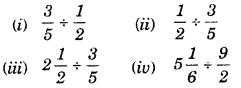Solution: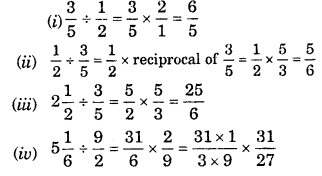Try These (Page 46):

Question 1.
Look at the following table and fill up the blank spaces :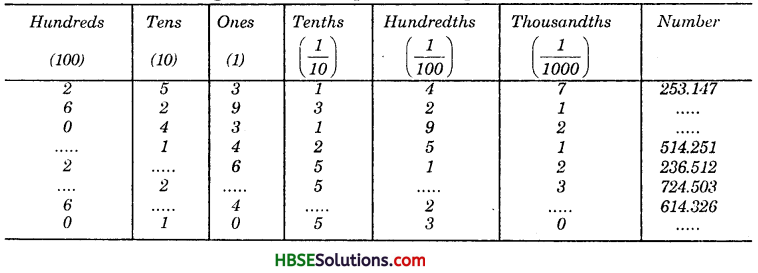Using this table you can write a decimal number in its expanded form also. For example 253.417
= 2 x 100 + 5 x 10 + 3 x 1 + 4 x ($$\frac{1}{10}$$) + 1 x ($$\frac{1}{100}$$) + 7 x ($$\frac{1}{1000}$$). So, a decimaln number and its expanded form can be written if the place values of the digits are known to us.
Solution: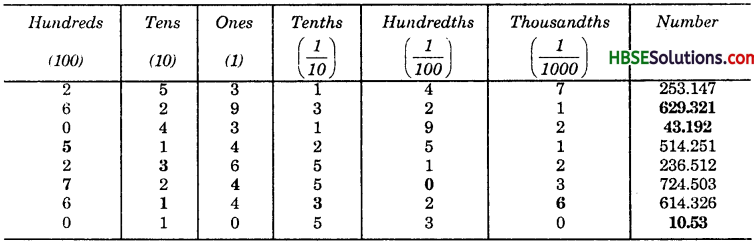Try These (Page 50):

Question 1.
1. Find: (i) 2.7×4
(ii) 1.8 x 1.2
(iii) 2.3 x 4.35
2. Arrange the products obtained in (1) in descending order.
Solution:
1.(i) 2.7 x 4 = 10.8
(ii) 1.8 x 1.2 = 2.16
(iii) 2.3 x 4.35 = 10.005
2. 10.8 > 10.005 > 2.16.

Try These (Page 51):

Question 1.
Find :
(i) 0.3 x 10
(ii) 1.2 x 100
(iii) 56.3 x 1000
Solution:
(i) 0.3 x 10 = 3
(ii) 1.2 x 100 = 120
(iii) 56.3 x 1000 = 56300

Try These (Page 53):

Question 1.
Find :
(i) 235.4 ÷ 10
(ii) 235.4 ÷ 100
(iii) 235.4 ÷ 1000
Solution:
(i) $$\frac{235.4}{10}$$= 23.54
(ii) $$\frac{235.4}{100}$$ = 2.354
(iii) $$\frac{235.4}{1000}$$ = 0.2354Try These (Page 53):

Question 1.
Find : (i) 35.7 ÷ 3 = ?
(ii) 25.5 ÷ 3 = ?
Solution:
(i) 35.7 ÷ 3 = 11.9,
(ii) 25.5 ÷ 3 = 8.5

Try These (Page 53):

Question 1.
Find : (i) 43.15 ÷ 5 = ?
(ii) 82.44 ÷ 6 = ?
Solution:
(i) 43.15 ÷ 5 = 8.63,
(ii) 82.44 ÷ 6 = 13.74

Try These (Page 53):

Question 1.
Find : (i) 15.5 ÷ 5 (ii) 126.35 ÷ 7
Solution:
(i) $$\frac{15.5}{5}$$ = 3.1
(ii) $$\frac{126.35}{7}$$ = 18.05Try These (Page 54):

Question 1.
Find
(i) $$\frac{7.75}{0.25}$$
(ii) $$\frac{42.8}{0.02}$$
(iii) $$\frac{5.6}{1.4}$$
Solution:
(i) $$\frac{7.75}{0.25}=\frac{775}{25}$$ = 31
(ii) $$\frac{42.8}{0.02}=\frac{4280}{2}$$ = 2140
(iii) $$\frac{5.6}{1.4}=\frac{56}{14}$$ = 4